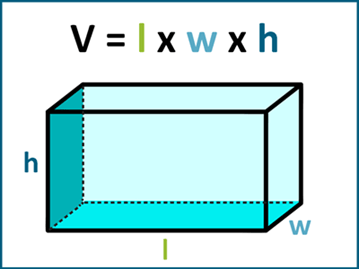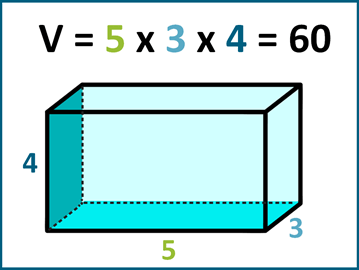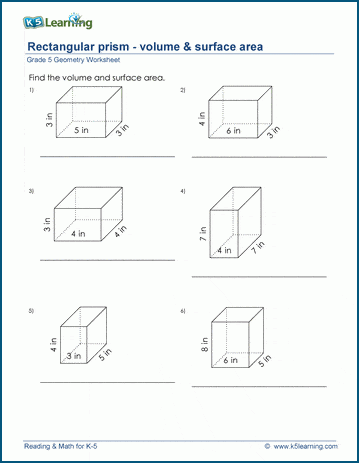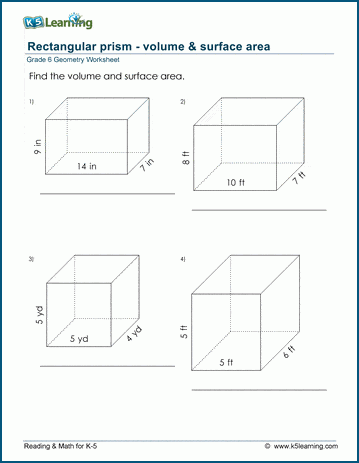# How to Find the Volume of Rectangular Prisms

## Volume formula for rectangular prisms

The volume of a rectangular prism is given by the formula:

V = l x w x h

where V is the volume, l is the length, w is the width, and h is the height.## How to calculate the volume of a rectangular prism

Here's an example:

Suppose we have a rectangular prism with the following dimensions:

Length (l) = 5 cm

Width (w) = 3 cm

Height (h) = 4 cm

To find the volume, we use the formula:

V = l x w x h = 5 cm x 3 cm x 4 cm = 60 cubic cm

Therefore, the volume of the rectangular prism is 60 cubic cm.Note that the units for volume are cubic units (e.g. cubic cm, cubic inches, etc.).

## Grade 5 volume and surface area of rectangular prisms worksheets

In our worksheets, we combine the practice of calculating the volume and surface area of rectangular prisms. Here’s the set for grade 5.## Volume and surface area of rectangular prisms practice for grade 6

This is the set of worksheets for grade 6 for finding the volume and surface areas of rectangular prisms.Become a Member

This content is available to members only.